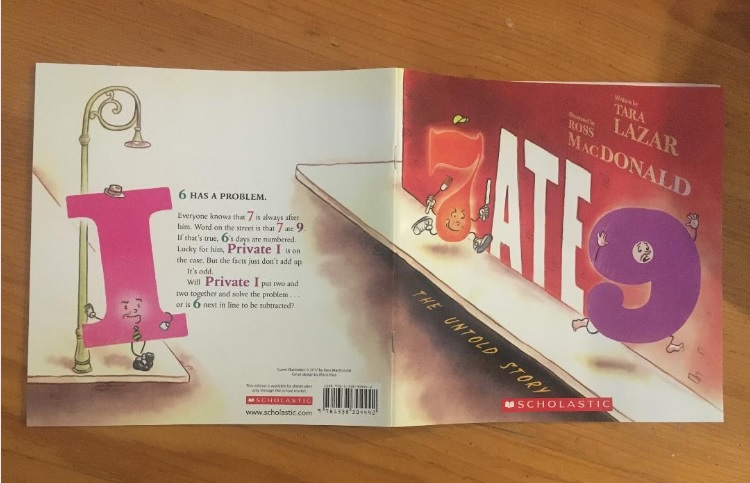# 1656 Seven Ate Nine: Puzzle and a Picture Book

Contents

### Today’s Puzzle:

Today’s Puzzle is a relatively easy level 6 puzzle with consecutive numbers 7, 8, and 9 prominent among the clues. Write the numbers 1 to 10 in both the 1st column and the top row so that those numbers and all the given clues work together to make a multiplication table. Will the common factor of 24 and 32 be 4 or 8? Will 20 and 12’s common factor be 2 or 4? Don’t guess! Look at the other clues. They all work together to help you find a logical way to solve the puzzle.### The Book Seven Ate Nine:

I ordered several books from my granddaughter’s book order. One of those books was Seven Ate Nine, a delightful tale whose characters are numbers and letters. The back cover summaries the story, “6 has a problem. Everyone knows that 7 is always after him. Word on the street is that 7 ate 9. If that’s true, 6’s days are numbered. Lucky for him, Private I is on the case. But the facts just don’t add up. It’s odd. Will Private I put two and two together and solve the problem . . . or is 6 next in line to be subtracted?”My preschool grandchildren loved listening to this story. It is filled with math puns and surprising twists and turns. Other than familiarity with the concept of counting, mathematical understanding is not a prerequisite to following the story. Older kids and even adults will enjoy references to several mathematical concepts including odd, even, addition, subtraction, multiplication, division, doubling, measurement, positives, negatives, and pi.

### Factors of 1656:

• 1656 is a composite number.
• Prime factorization: 1656 = 2 × 2 × 2 × 3 × 3 × 23, which can be written 1656 = 2³ × 3² × 23.
• 1656 has at least one exponent greater than 1 in its prime factorization so √1656 can be simplified. Taking the factor pair from the factor pair table below with the largest square number factor, we get √1656 = (√36)(√46) = 6√46.
• The exponents in the prime factorization are 3, 2, and 1. Adding one to each exponent and multiplying we get (3 + 1)(2 + 1)(1 + 1) = 4 × 3 × 2 = 24. Therefore 1656 has exactly 24 factors.
• The factors of 1656 are outlined with their factor pair partners in the graphic below.### More About the Number 1656:

1656 is the difference of two squares SIX different ways:
415² – 413² = 1656,
209² – 205² = 1656,
141² – 135² = 1656,
75² – 63² = 1656,
55² – 37² = 1656, and
41² – 5² – = 1656.
That last one means we are only 25 numbers away from the next perfect square, 1681.

## 2 thoughts on “1656 Seven Ate Nine: Puzzle and a Picture Book”

1.Rose

That book sounds awesome! We’ve told our grandchildren the joke, ‘why was 6 afraid of 7? Because 7,8,9.’ We laugh, but the grandkids might be getting too old for our humor. Maybe I’ll have to see if they can solve some of your puzzles. I really enjoy your site, it stretches my brain. 😊

•ivasallay

I like the kind of jokes you tell! I’m so glad you like the site, and I hope your grandkids will enjoy the puzzles. They still might get a kick out of the book. https://m.youtube.com/watch?v=3a03HEBWvbU

This site uses Akismet to reduce spam. Learn how your comment data is processed.